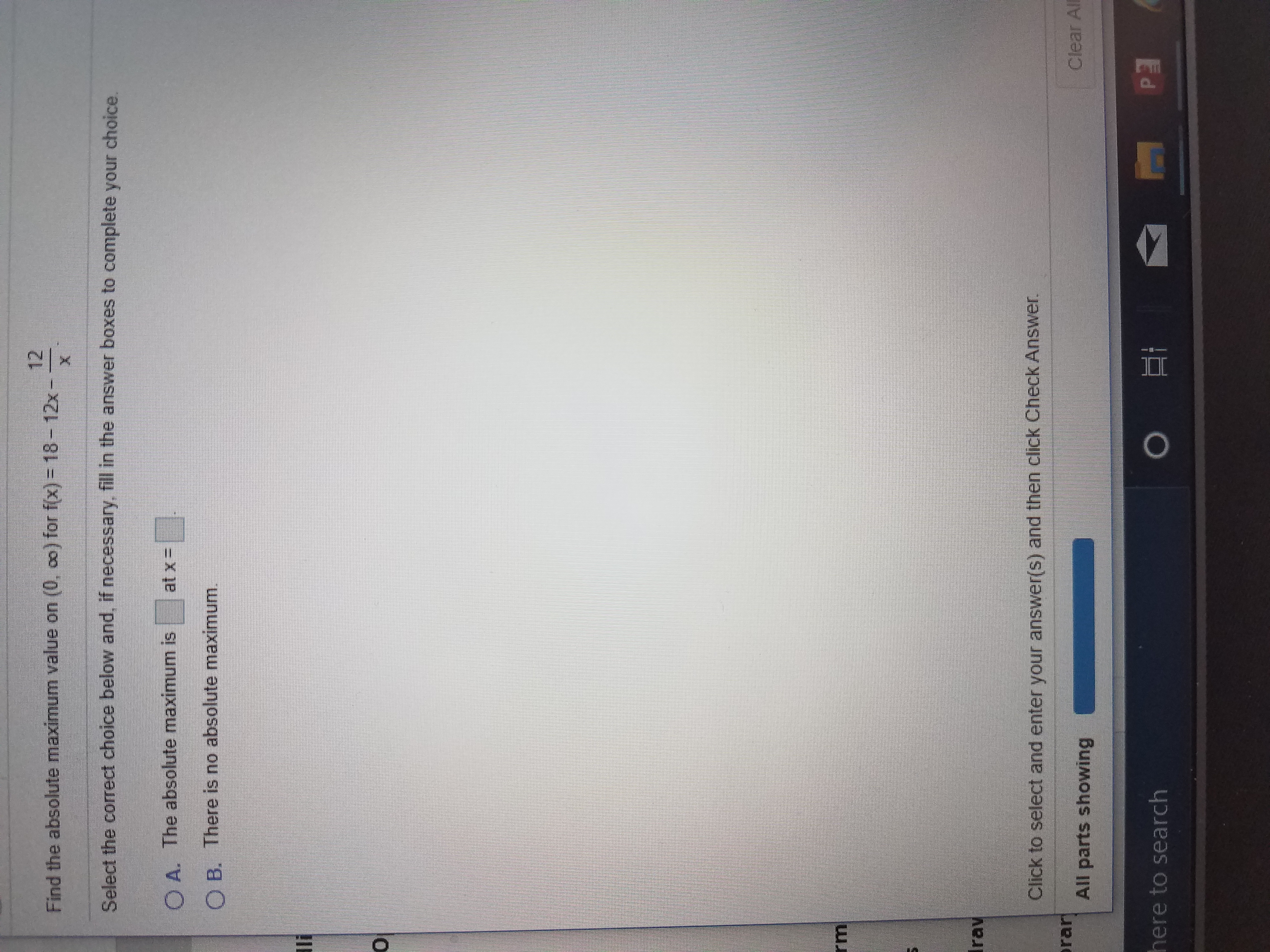# 12Find the absolute maximum value on (0, co) for f(x) = 18-12x-XSelect the correct choice below and, if necessary, fill in the answer boxes to complete your choice.at x-O A. The absolute maximum isO B. There is no absolute maximum.lirmIravClick to select and enter your answer(s) and then click Check Answer.rarAll parts showingClear Allhere to search

Question
21 viewshelp_outlineImage Transcriptionclose12 Find the absolute maximum value on (0, co) for f(x) = 18-12x- X Select the correct choice below and, if necessary, fill in the answer boxes to complete your choice. at x- O A. The absolute maximum is O B. There is no absolute maximum. li rm Irav Click to select and enter your answer(s) and then click Check Answer. rar All parts showing Clear All here to search fullscreen
check_circle

star
star
star
star
star
1 Rating
Step 1

f(x) = 18 - 12x - 12/x

f'(x) = -12 + 12 / x2

Step 2

The critical points are given by f'(x) = -12 + 12 / x2 = 0

Hence,  12 / x2 = 12

Hence, x2 = 1

Hence, x = 1 or -1

However the given interval for x is (0, ∞)

x = -1 falls outside this interval, hence  x = 1 is the only critical point in the given interval.

Step 3
Hence the critical point is x = 1 and the end points of the interval are x = 0 and

f(0) = 18 - 12 x 0 - 12 / 0 = -∞

f(1) = 18 - 12 x 1 - 12 / 1 = -6

f(∞) = 18 - 12 x ∞ - 12 / ∞ = -∞

Since, f(1) is the highest value, hence, the absolute maxima occurs at x = 1 and the maxima is -6

...

### Want to see the full answer?

See Solution

#### Want to see this answer and more?

Solutions are written by subject experts who are available 24/7. Questions are typically answered within 1 hour.*

See Solution
*Response times may vary by subject and question.
Tagged in

### Derivative